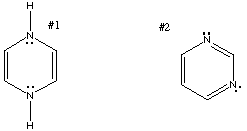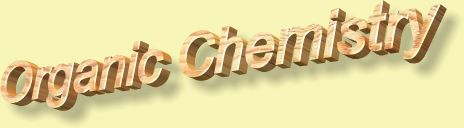1.* (1997 1 1) Look over the following names which designate various organic molecules. BEWARE, some of these names may not be correct. For those names that are correct, write a line bond structure in the space beside the name. If the name is incorrect, write a brief statement explaining what is wrong.

A. 2-pentanone

C. 2-hexanal

D. methyl formate

E. trans-1,2-dichlorocyclobutane

F. phenyl-isopropyl ether

G. acetonitrile

2. Five and six-membered rings of carbon atoms are favored (i.e. more stable) over rings that have other numbers of atoms. Explain why.

3.* (1997 1 2) Below is a line drawing of imidazole, an aromatic heterocycle found in the amino acid histidine.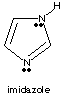A. Draw an arrow to each nitrogen and indicate the hybridization state (sp3, sp2, or sp) for that nitrogen.

B. Each nitrogen has an electron pair. For each nitrogen indicate the nature of the orbital containing that electron pair.

C. Explain the origin of imidazole’s aromaticity.

D. Imidizole is a Brønsted base; protonation of imidizole forms a cation, C3H5N+. Complete the partial structure of the cation, showing line bonds and electron pairs.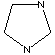4.* (1997 1 4) Write a structural formula for the smallest hydrocarbon (only C and H atoms) which manifests every hybridization state of carbon.

5. Which has the shorter carbon-carbon bond length: allene or ethylene? Why?

6.* (1996 F 8) Pattern recognition: place the letter corresponding to the correct structure in the space under the formula.

A. a steroid
B. a penicillin
C. a carbohydrate
D. a high explosive
E. the essence of garlic
F. a prostaglandin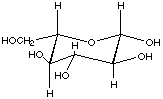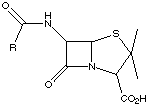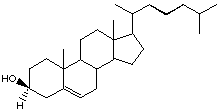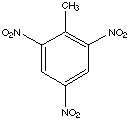7.* (1995 1 1) Write structural formulas for an example of each of the following:

A. The smallest ketone with 2 sites of unsaturation:

B. A b-lactone

C. An anti-aromatic hydrocarbon

D. The molecule called formamide

G. The simplest, naturally occurring , chiral amino acid.

8. Consider the molecule shown below.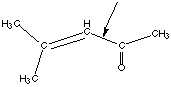A. Briefly explain why rotation about the labeled carbon-carbon bond is hindered.

B. Briefly explain why cumulated systems (such as allene) are inherently unstable.

9.* (1995 1 2) Consider a-isomethylionone, whose structural formula is shown below: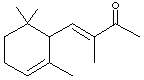A. How many sites of unsaturation are in a-isomethylionone?

B. How many sites of unsaturation are in the hydrogenation product of this molecule? The molecular formula of the hydrogenation product is C14H26O.

10.* (1995 1 3) Consider a hydrocarbon X, (molecular formula C5H8), which is chiral. This substance manifests all three hybridization states of carbon. Reaction of X with H2 over a Pd metal catalyst forms n-pentane. Such reactions do not break carbon-carbon bonds.

A. How many sites of unsaturation are in X?

B. Draw a three dimensional structural formula for X.

11. Draw resonance structures for the following molecules. Label the hybridization states and formal charges for each structure you draw.

A. Carbon dioxide (CO2)

B. Carbonate ion (CO32-)

C. Nitrite ion (NO21-)

D. Ozone (O3)

12.* (1994 1 3) The names of three nitrogen containing compounds are given below. Under each write a line-bond structural formula, show non-bonded valence electron pairs and indicate the hybridization state of nitrogen.

A. triethylamine

B. acetonitrile (or cyanomethane)

C. formamide

13.* (1994 1 5) The reactive compound 1,4-dibromobuta-1,2,3-triene has the molecular formual C4H2Br2.

Show line-bond structures, hybridization state of each carbon, and shape of the molecule. Indicate whether the molecule is chiral.

14. A molecule that is known as a "proton sponge" is pictured below. Note that the lone pairs on the nitrogen atoms are in orbitals that are in the plane of the molecule.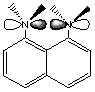A. Is the proton sponge aromatic?

B. Why does this molecule suck up protons so well?

C. Do you think the proton sponge would be as effective if it had protons replacing its methyl groups? Why or why not?

15.* (1993 F 2) Using molecular formulas, illustrate specific examples of each of the following.

A. A diamagnetic, neutral molecule isoelectronic with the nitrite anion, NO2-:

B. An aromatic compound which contains no carbon:

16.* (1993 1 3) Use structural (line bond) formulas to illustrate the following:

A. ethanol

C. propanal

D. vinyl acetate

17. Consider the molecule of borazine shown below.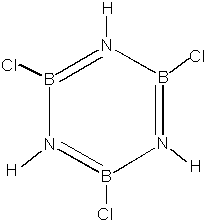A. Label the formal charges and hybridization states of all of the atoms in borazine.

B. Where do the electrons in borazine's pi electron cloud come from? Is borazine aromatic?

C. What stable resonance structures are possible for borazine?

D. Which would be more stable: borazine or benzene? Briefly explain your answer.

18.* (1993 1 4)

A. What is meant by an "antiaromatic" compound? Write a standard structural formula for any example of such a substance.

B. Two of the following three substances have something in common which is reflected in their structures. Can you identify this commonality? Propose structures for all three: O3, CO2, NO2-.

19.* (1992 1 1) Consider the molecule shown below.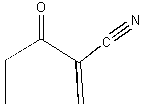A. How many sites of unsaturation does this molecule contain?

B. Is this substance chiral?

C. Label the hybridization state of all of the carbon atoms in this molecule.

D. Label the functional groups in this molecule.

20.* (1992 1 3) Consider the following 3 molecules:

CH3CH2NH2, CH3CONH2, CH3CN.

A. Which one has the longest carbon-nitrogen bond? Explain, using hybridization arguments.

21.* (1992 1 5) Taxol is an exciting anticancer agent.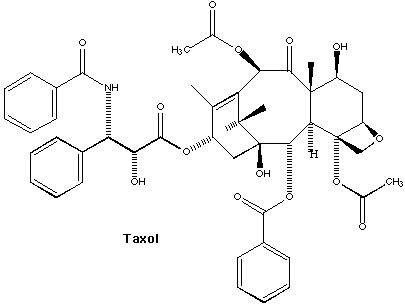Next to each functional group in the drawing above, write the number of the appropriate functional group.

 1) ene 7) thiol 13) anhydride 2) arene 8) oic acid 14) amide 3) yne 9) al 15) lactam 4) ol 10) one 16) nitrile 5) ether 11) ester 17) imide 6) amine 12) lactone

22. For the names that are incorrect or ambiguous, explain why. For those that are correct, draw the structure.

A. 1,2-dibromocyclobutane

B. trinitrotoluene

C. 3,3 dichlorobenzene

D. 1-pentanone

E. diethylcarbonate

F. propanedial

23.* (1991 1 2) Some of the following names are wrong. For those that are, explain why. For those that are correct, propose a structural formula.

A. 1,4-propanediol

B. 2-butanal